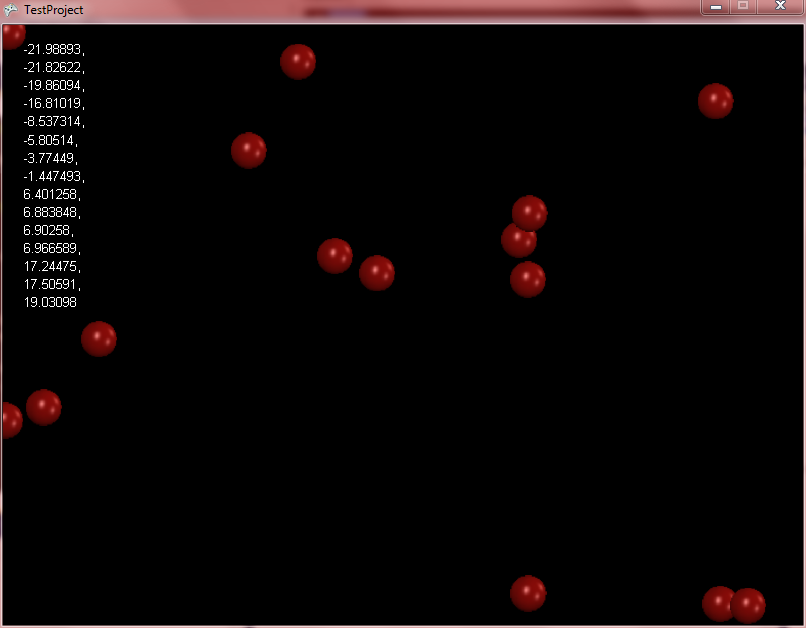# FlatRedBall.Math.PositionedObjectList.GetFirstAfter

## Introduction

The GetFirstAfter value can be used to get the index of the next object after a given position value. For example, if you are working with a group of Circles and are implementing some spacial partitioning logic, you can use GetFirstAfter to find the index of the first Circle in a list to begin your loop for partitioning.

## Code Example

Assuming you have an already-sorted list of PositionedObjects, and that mPositionedObjectList is a valid PositionedObjectList, the following method will return the first index of any PositionedObject in the list after X = 0.

```float xToLookAfter = 0;
Axis axis = Axis.X;
int lowBound = 0;
int highBound = mSprites.Count;
int indexOfObject = mSprites.GetFirstAfter(xToLookAfter, axis, lowBound, highBound);
```

## Inserting in sorted lists

GetFirstAfter is used in already-sorted lists, and it is usually used to insert new items in a PositionedObjectList such that the PositionedObjectList remains sorted after the insertion. The following code can be used to create 15 Sprites which will be inserted in order of their X value:

```using FlatRedBall.Math;
```

Add the following to Initialize after initializing FlatRedBall:

```string valueRecord = "";
PositionedObjectList<Sprite> sortedList = new PositionedObjectList<Sprite>();

for (int i = 0; i < 15; i++)
{
SpriteManager.Camera.PositionRandomlyInView(sprite, 40, 40);

int indexToInsertAt = sortedList.GetFirstAfter(sprite.X, Axis.X, 0, sortedList.Count);
sortedList.Insert(indexToInsertAt, sprite);
}

for (int i = 0; i < sortedList.Count; i++)
{
if (i != 0)
{
valueRecord += ",\n";
}
valueRecord += sortedList[i].X;
}

FlatRedBall.Debugging.Debugger.CommandLineWrite(valueRecord);
```